# Geometric Deep Learning Extension Library for PyTorch

##### Overview

PyTorch Geometric (PyG) is a geometric deep learning extension library for PyTorch.

It consists of various methods for deep learning on graphs and other irregular structures, also known as geometric deep learning, from a variety of published papers. In addition, it consists of an easy-to-use mini-batch loader for many small and single giant graphs, multi gpu-support, a large number of common benchmark datasets (based on simple interfaces to create your own), and helpful transforms, both for learning on arbitrary graphs as well as on 3D meshes or point clouds. Click here to join our Slack community!

PyTorch Geometric makes implementing Graph Neural Networks a breeze (see here for the accompanying tutorial). For example, this is all it takes to implement the edge convolutional layer:

```import torch
from torch.nn import Sequential as Seq, Linear as Lin, ReLU
from torch_geometric.nn import MessagePassing

class EdgeConv(MessagePassing):
def __init__(self, F_in, F_out):
super(EdgeConv, self).__init__(aggr='max')  # "Max" aggregation.
self.mlp = Seq(Lin(2 * F_in, F_out), ReLU(), Lin(F_out, F_out))

def forward(self, x, edge_index):
# x has shape [N, F_in]
# edge_index has shape [2, E]
return self.propagate(edge_index, x=x)  # shape [N, F_out]

def message(self, x_i, x_j):
# x_i has shape [E, F_in]
# x_j has shape [E, F_in]
edge_features = torch.cat([x_i, x_j - x_i], dim=1)  # shape [E, 2 * F_in]
return self.mlp(edge_features)  # shape [E, F_out]```

In detail, the following methods are currently implemented:

Head over to our documentation to find out more about installation, data handling, creation of datasets and a full list of implemented methods, transforms, and datasets. For a quick start, check out our examples in the `examples/` directory.

If you notice anything unexpected, please open an issue and let us know. If you have any questions or are missing a specific feature, feel free to discuss them with us. We are motivated to constantly make PyTorch Geometric even better.

## Installation

We provide pip wheels for all major OS/PyTorch/CUDA combinations, see here.

### PyTorch 1.8.0

To install the binaries for PyTorch 1.8.0, simply run

```\$ pip install torch-scatter -f https://pytorch-geometric.com/whl/torch-1.8.0+\${CUDA}.html
\$ pip install torch-sparse -f https://pytorch-geometric.com/whl/torch-1.8.0+\${CUDA}.html
\$ pip install torch-cluster -f https://pytorch-geometric.com/whl/torch-1.8.0+\${CUDA}.html
\$ pip install torch-spline-conv -f https://pytorch-geometric.com/whl/torch-1.8.0+\${CUDA}.html
\$ pip install torch-geometric```

where `\${CUDA}` should be replaced by either `cpu`, `cu101`, `cu102`, or `cu111` depending on your PyTorch installation.

`cpu` `cu101` `cu102` `cu111`
Linux
Windows
macOS

### PyTorch 1.7.0/1.7.1

To install the binaries for PyTorch 1.7.0 and 1.7.1, simply run

```pip install torch-scatter -f https://pytorch-geometric.com/whl/torch-1.7.0+\${CUDA}.html
pip install torch-sparse -f https://pytorch-geometric.com/whl/torch-1.7.0+\${CUDA}.html
pip install torch-cluster -f https://pytorch-geometric.com/whl/torch-1.7.0+\${CUDA}.html
pip install torch-spline-conv -f https://pytorch-geometric.com/whl/torch-1.7.0+\${CUDA}.html
pip install torch-geometric```

where `\${CUDA}` should be replaced by either `cpu`, `cu92`, `cu101`, `cu102`, or `cu110` depending on your PyTorch installation.

`cpu` `cu92` `cu101` `cu102` `cu110`
Linux
Windows
macOS

Note: Binaries of older versions are also provided for PyTorch 1.4.0, PyTorch 1.5.0 and PyTorch 1.6.0 (following the same procedure).

### From master

In case you want to experiment with the latest PyG features which did not get released yet, you can install PyG from master via

``````pip install git+https://github.com/rusty1s/pytorch_geometric.git
``````

## Running examples

``````cd examples
python gcn.py
``````

## Cite

Please cite our paper (and the respective papers of the methods used) if you use this code in your own work:

``````@inproceedings{Fey/Lenssen/2019,
title={Fast Graph Representation Learning with {PyTorch Geometric}},
author={Fey, Matthias and Lenssen, Jan E.},
booktitle={ICLR Workshop on Representation Learning on Graphs and Manifolds},
year={2019},
}
``````

Feel free to email us if you wish your work to be listed in the external resources.

## Running tests

``````python setup.py test
``````
• #### Issue reproducing the results of the original ecc implementation. Pooling layer and conv layer are giving different results of the original implementation

As I mentioned in #319 I have problems to reproduce the ecc implemenation using pytorch_geometric. I found some differences between the results obtained, first one is that the results of both convolution operations using the same weights have different results. Moreover, the results of the pooling layers are also different.

I created a test that checks this things. Basically, the scripts load the same weights to both implementations. These weights are obtained from train a network using the ecc_implementation. Below you can see the output of my test.

``````ECC Weights and PyGeometric weights are equal: True #I am only doing a re-check in order to be sure that both weights are equal before to load to the models.
Starting validation:
ecc features conv1:  (997, 16) #Shape of the output of first conv in ecc implementation
pygeometric features conv1:  (997, 16) #Shape of the output of first conv in pygeometric implementation
Max difference between features of first conv 2.549824
Output of ecc pooling:  (398, 32)
Output of PyGeometric pooling:  (385, 32)
Pygeomtric Acc:  41.51982378854625  Ecc accuracy:  63.65638766519823
Pygeomtric Loss:  2.435516586519023  Ecc Loss:  0.9878960176974138
``````

As you can observe this difference has an impact to the accuracy using the same weights. You can find the source code here. One important thing, the data used for this tests is obtained from the original code of the ecc.

opened by dhorka 93

## ❓ Questions & Help

I tried to use pytorch model with `MessagePassing` layer in C++ code. As described in pytorch_geometric documentation, I generate torch model with my own MP layer and successfully convert the model.

But in the process of executing C++ code, I face the error like below:

``````Unknown builtin op: torch_sparse::ptr2ind.
Could not find any similar ops to torch_sparse::ptr2ind. This op may not exist or may not be currently supported in TorchScript.
:
File "/home/sr6/kyuhyun9.lee/env_ML/lib/python3.6/site-packages/torch_sparse/storage.py", line 166
rowptr = self._rowptr
if rowptr is not None:
row = torch.ops.torch_sparse.ptr2ind(rowptr, self._col.numel())
~~~~~~~~~~~~~~~~~~~~~~~~~~~~~~ <--- HERE
self._row = row
return row
Serialized   File "code/__torch__/torch_sparse/storage.py", line 825
if torch.__isnot__(rowptr, None):
rowptr13 = unchecked_cast(Tensor, rowptr)
row15 = ops.torch_sparse.ptr2ind(rowptr13, torch.numel(self._col))
~~~~~~~~~~~~~~~~~~~~~~~~ <--- HERE
self._row = row15
_150, _151 = True, row15
'SparseStorage.row' is being compiled since it was called from 'SparseStorage.__init__'
File "/home/sr6/kyuhyun9.lee/env_ML/lib/python3.6/site-packages/torch_sparse/storage.py", line 133
if not is_sorted:
idx = self._col.new_zeros(self._col.numel() + 1)
idx[1:] = self._sparse_sizes * self.row() + self._col
~~~~~~~~ <--- HERE
if (idx[1:] < idx[:-1]).any():
perm = idx[1:].argsort()
Serialized   File "code/__torch__/torch_sparse/storage.py", line 267
idx = torch.new_zeros(self._col, [_29], dtype=None, layout=None, device=None, pin_memory=None)
_30 = (self._sparse_sizes)
_31 = torch.add(torch.mul((self).row(), _30), self._col, alpha=1)
~~~~~~~~~~ <--- HERE
_32 = torch.slice(idx, 0, 1, 9223372036854775807, 1)
_33 = torch.copy_(_32, _31, False)
'SparseStorage.__init__' is being compiled since it was called from 'GINLayerJittable_d54f76.__check_input____1'
Serialized   File "code/__torch__/GINLayerJittable_d54f76.py", line 40
pass
return the_size
def __check_input____1(self: __torch__.GINLayerJittable_d54f76.GINLayerJittable_d54f76,
~~~~~~~~~~~~~~~~~~~~~~~~~~~~~~~~~~~~~~~~~~~~~~~~~~~~~~~~~~~~~~~~~~~~~~~~~~~~~~~~~~~...  <--- HERE
edge_index: __torch__.torch_sparse.tensor.SparseTensor,
size: Optional[Tuple[int, int]]) -> List[Optional[int]]:

Aborted (core dumped)

``````

Since I have no experience of pytorch jit, I cannot find any clue to solve this. How can I handle this error?

bug feature help wanted
opened by Nanco-L 32
• #### Segmentation Fault in Forward Loop of Edge Conv

Hey there!

I'm testing out edge conv and am running into some issues. I'm getting a segmentation fault during the knn_graph generation:

``````line 13: 14361 Segmentation fault      (core dumped) CUDA_VISIBLE_DEVICES="\$gpuNum" python ...
``````

Here's output using pysnooper, I traced the error to this location:

``````Starting var:.. batch = None
Starting var:.. pos = tensor([[-3.1472e-01, -7.1309e-01, -1.5181e-01,  1.3493e+00,  1.0879e+00,          4.9691e-01, -1.54...
Starting var:.. self = Net(  (conv1): EdgeConv(nn=Sequential(    (0): Linear(in_features=50, out_features=64, bias=True)   ...
17:39:03.629809 call        41  def forward(self, pos, batch):
17:39:03.680347 line        42          edge_index = knn_graph(pos, k=20, batch=batch)
~
``````

Last line is where error is happening.

As more context, I'm generating my point cloud using a CNN, batch and positions are shown above, cannot seem to make it through the generation of the edge index.

opened by jlevy44 31

### 🐛 Describe the bug

Hi. I am a computational physics researcher and was using PyG very well. my pyg code was working well a few weeks ago, but now that I run my code, it is not working anymore without any changes.

the problem is like below. I have many material structures and in my "custom_dataset" class, these are preprocessed and all graph informations (node features, edge features, edge index etc) are inserted into "Data" object in PyTorch geometric. You can see that each preprocessed sample with index \$i\$ was printed normal "Data" object in pyg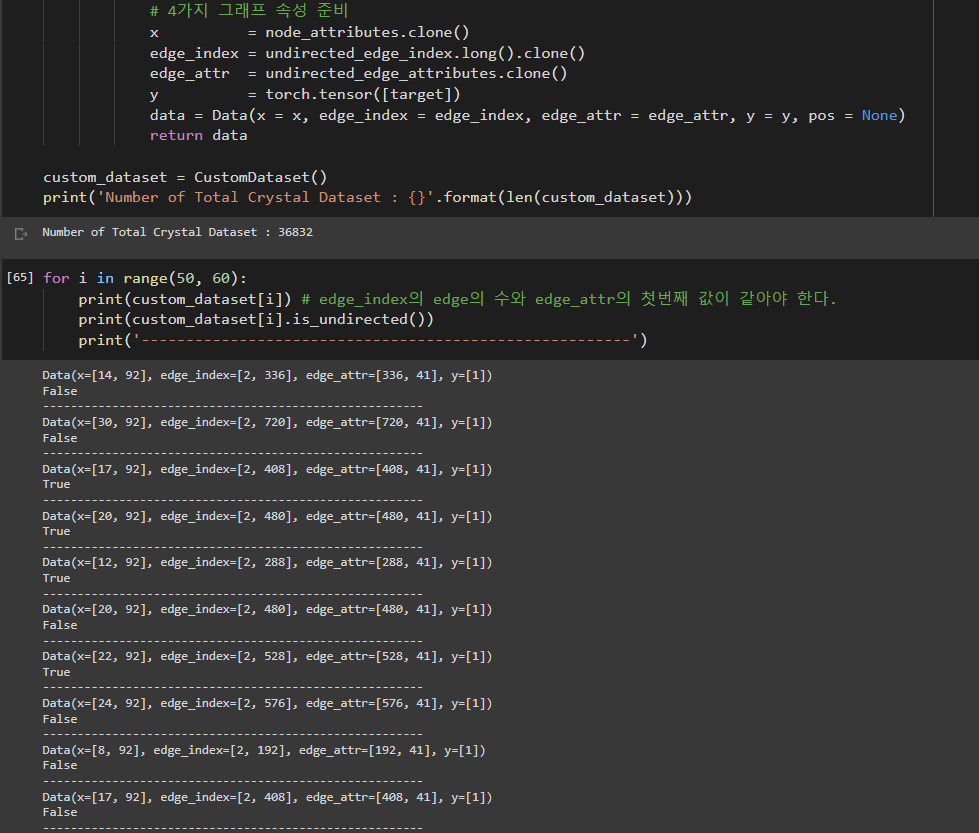But When I insert my custom dataset class into pyg DataLoader and I did like below,

``````sample = next(iter(train_loader)) # batch sample
``````

batch sample is denoted by "DataDataBatch". I didn't see this kind of object name. and i can't use "sample.x' or "sample.edge_index" command. Instead I need to do like this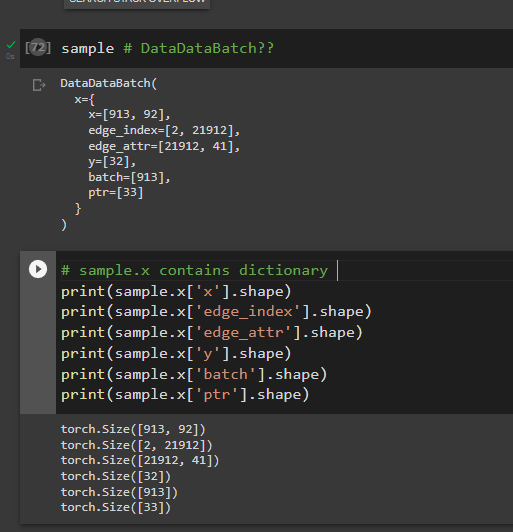I want to use expressions like "sample.x", "sample.edge_index" or "sample.edge_attr" as like before. I expect your kind explanations. Thank you.

### Environment

• PyG version: `2.0.5`
• PyTorch version: `1.11.0+cu113`
• OS: `GoogleColab Pro Plus`
• Python version: `Python 3.7.13 in colab`
• CUDA/cuDNN version:
• How you installed PyTorch and PyG (`conda`, `pip`, source):
``````# Install required packages.
import os
import torch
os.environ['TORCH'] = torch.__version__
print(torch.__version__)
!pip install -q torch-scatter -f https://data.pyg.org/whl/torch-\${TORCH}.html
!pip install -q torch-sparse -f https://data.pyg.org/whl/torch-\${TORCH}.html
!pip install -q git+https://github.com/pyg-team/pytorch_geometric.git
!pip install -q pymatgen==2020.11.11
``````
• Any other relevant information (e.g., version of `torch-scatter`):
bug
opened by Amadeus-System 29

### 🚀 The feature, motivation and pitch

Currently, `NeighborLoader` is designed to be applied in node-level tasks and there exists no option for mini-batching in link-level tasks.

To achieve this, users currently rely on a simple but hacky workaround, first utilized in `ogbl-citation2` in this example.

The idea is straightforward and simple: For `input_nodes`, we pass in both the source and destination nodes for every link we want to do link prediction on (both positive and negative):

``````loader = NeighborLoader(data, input_nodes=edge_label_index.view(-1), ...)
``````

Nonetheless, PyG should provide a dedicated class to perform mini-batch on link-level tasks, re-using functionality from `NeighborLoader` under-the-hood. An API could look like:

``````class LinkLevelNeighborLoader(
data,
input_edges=...
input_edge_labels=...
with_negative_sampling=True,
**kwargs,
)
``````

NOTE: This workaround currently only works for homogenous graphs!

@RexYing @JiaxuanYou

feature 0 - Priority P0
opened by rusty1s 28
• #### [WIP] MessagePassing.jittable()

This is a new feature pursuant to issue #997.

Difficulty -> the MessagePassing base class is highly dynamic (and really useful) and relies heavily on kwargs parsing to do its job. This means that it is manifestly not jittable, resulting in having to duplicate models and code outside of PyG if you want to run these models as a service (on nVidia TRITON or similar).

However, once a convolutional operation is written and input/output widths are defined its control flow is known. Therefore you can synthesize a concrete implementation of the control flow from the user's written model and an appropriate adaptation of the propagate() function of the MessagePassing base class. This is achieved using object inheritance and dynamic loading of python modules based on synthesized code.

This use of this functionality proceeds right now as follows:

1. write your convolution op (`MyConvOp`)+ some model (`MyNet`) that uses it
2. instead of `self.conv1 = MyConvOp(params)` you do:
``````   conv1_class = MyConvOp(params).jittable(args_to_forward)
self.conv1 = conv1_class(params)
``````

where `args_to_forward` are the parameters you would normally pass to forward.

3. `model = torch.jit.script(MyNet())`

I would like to make it so that 2 works without needing example data, but it was the simplest way to implement a partial trace.

This PR is functional and tests for all existing conv models are being extended to include jitting tests. So far each conv layer has needed a little tweaking to make it jit compatible, typically just adding a few type annotations. Occasionally some more type-static code needs to be re-written in the layer.

Checklist of conv layers tested for with jitting functionality in CI:

• [x] Basic test in test/nn/conv/test_message_passing.py
• [x] AGNNConv
• [x] APPNP
• [ ] ARMAConv
• [x] CGConv
• [x] ChebConv
• [x] DNAConv
• [x] EdgeConv
• [ ] DynamicEdgeConv - NB: this needs to wait on https://github.com/rusty1s/pytorch_cluster/pull/42
• [x] FeaStConv
• [x] GATConv
• [x] GatedGraphConv
• [x] GCNConv
• [x] GINConv
• [x] GINEConv
• [x] GMMConv
• [x] GraphConv
• [ ] GravNetConv - NB: this needs to wait on https://github.com/rusty1s/pytorch_cluster/pull/42
• [ ] HypergraphConv
• [x] NNConv
• [x] PointConv
• [x] PPFConv
• [x] RGCNConv
• [x] SAGEConv
• [x] SGConv
• [x] SignedConv
• [x] SplineConv
• [x] TAGConv
• [ ] XConv - NB: this needs to wait on https://github.com/rusty1s/pytorch_cluster/pull/42
opened by lgray 28

## 📚 Installation

I am able to install torch-scatter using 'pip3 install torch-scatter'.

But it failed when I use 'conda install torch-scatter' and 'pip install torch-scatter'

By using pip3, I cannot install torch-scatter to my anaconda3 environment. Then, when I run the examples/code, I aways get 'PackagesNotFoundError' for torch-scatter

## Environment

• OS: macOS
• Python version: 10.14.5
• PyTorch version: 1.1.0
• CUDA/cuDNN version: NO
• GCC version: 4.2.1
• How did you try to install PyTorch Geometric and its extensions (pip, source): I tried pip, and it is not success. Then, I changed to pip3, it is working. But I still get module not found error when I run code/example. And I cannot install using 'conda install torch-scatter'
• Any other relevant information:

## Checklist

• [x] I followed the installation guide.
• [ ] I cannot find my error message in the FAQ.
• [ ] I set up CUDA correctly and can compile CUDA code via `nvcc`.
• [ ] I have cloned the repository and tried a manual installation from source.
• [ ] I do have multiple CUDA versions on my machine.
• [ ] I checked if the official extension example runs on my machine.
• [ ] The offical extension example runs on my machine.

## Error logs:

Thank you very much!

opened by Shane-2015 28

## 🐛 Bug

There seems to be a problem running the encoder.

``````data=graph.graph

gvae= VGAE(Encoder(data.num_features,5))

model=gvae

optimizer = torch.optim.Adam(model.parameters(), lr=0.01)

def train():
model.train()
print(x)
print(edge_index)
z = model.encode(x, edge_index)
print(z)
loss = model.recon_loss(model.decoder(z), data.train_pos_edge_index,
loss.backward()
optimizer.step()

def test(pos_edge_index, neg_edge_index):
model.eval()
z = model.encode(x, edge_index)
return model.test(model.decoder(z), pos_edge_index, neg_edge_index)

for epoch in range(1, 201):
train()
auc, ap = test(data.val_pos_edge_index, data.val_neg_edge_index)
print('Epoch: {:03d}, AUC: {:.4f}, AP: {:.4f}'.format(epoch, auc, ap))

auc, ap = test(data.test_pos_edge_index, data.test_neg_edge_index)
print('Test AUC: {:.4f}, Test AP: {:.4f}'.format(auc, ap))
``````

Got this error:

RuntimeError Traceback (most recent call last) in () 25 26 for epoch in range(1, 201): ---> 27 train() 28 auc, ap = test(data.val_pos_edge_index, data.val_neg_edge_index) 29 print('Epoch: {:03d}, AUC: {:.4f}, AP: {:.4f}'.format(epoch, auc, ap))

in train() 9 print(x) 10 print(edge_index) ---> 11 z = model.encode(x, edge_index) 12 print(z) 13 loss = model.recon_loss(model.decoder(z), data.train_pos_edge_index,

/anaconda2/envs/py36/lib/python3.6/site-packages/torch_geometric-1.0.3-py3.6.egg/torch_geometric/nn/models/autoencoder.py in encode(self, *args, **kwargs) 31 def encode(self, *args, **kwargs): 32 r"""Runs the encoder and computes latent variables for each node.""" ---> 33 return self.encoder(*args, **kwargs) 34 35 def decode(self, z):

/anaconda2/envs/py36/lib/python3.6/site-packages/torch/nn/modules/module.py in call(self, *input, **kwargs) 487 result = self._slow_forward(*input, **kwargs) 488 else: --> 489 result = self.forward(*input, **kwargs) 490 for hook in self._forward_hooks.values(): 491 hook_result = hook(self, input, result)

in forward(self, x, edge_index) 28 29 def forward(self, x, edge_index): ---> 30 x = F.relu(self.conv1(x, edge_index)) 31 return self.conv2(x, edge_index) 32

/anaconda2/envs/py36/lib/python3.6/site-packages/torch/nn/modules/module.py in call(self, *input, **kwargs) 487 result = self._slow_forward(*input, **kwargs) 488 else: --> 489 result = self.forward(*input, **kwargs) 490 for hook in self._forward_hooks.values(): 491 hook_result = hook(self, input, result)

/anaconda2/envs/py36/lib/python3.6/site-packages/torch_geometric-1.0.3-py3.6.egg/torch_geometric/nn/conv/gcn_conv.py in forward(self, x, edge_index, edge_weight) 94 edge_index, norm = GCNConv.norm(edge_index, 95 x.size(0), edge_weight, ---> 96 self.improved, x.dtype) 97 self.cached_result = edge_index, norm 98

/anaconda2/envs/py36/lib/python3.6/site-packages/torch_geometric-1.0.3-py3.6.egg/torch_geometric/nn/conv/gcn_conv.py in norm(edge_index, num_nodes, edge_weight, improved, dtype) 81 82 row, col = edge_index ---> 83 deg = scatter_add(edge_weight, row, dim=0, dim_size=num_nodes) 84 deg_inv_sqrt = deg.pow(-0.5) 85 deg_inv_sqrt[deg_inv_sqrt == float('inf')] = 0

/anaconda2/envs/py36/lib/python3.6/site-packages/torch_scatter/add.py in scatter_add(src, index, dim, out, dim_size, fill_value) 70 [2, 4, 4, 0, 0, 0]]) 71 """ ---> 72 src, out, index, dim = gen(src, index, dim, out, dim_size, fill_value) 73 return out.scatter_add_(dim, index, src)

/anaconda2/envs/py36/lib/python3.6/site-packages/torch_scatter/utils/gen.py in gen(src, index, dim, out, dim_size, fill_value) 9 index_size = list(repeat(1, src.dim())) 10 index_size[dim] = src.size(dim) ---> 11 index = index.view(index_size).expand_as(src) 12 13 # Generate output tensor if not given.

RuntimeError: shape '' is invalid for input of size 307

opened by jlevy44 28
• #### Unpooling layers and generative architectures

Hey there! Thanks for your hard work with this project, it's super cool. In the end of the splineCNN paper, it was mentioned that unpooling layers were a future possibility. I'd love to try generating graphs from a continuous latent space. Any ideas for where I might start? Any projects like this one that you're aware of with generative capabilities? Thanks.

feature
opened by robclouth 27
• #### ERROR: No matching distribution found for torch-spine-conv

Hello, I am trying to install the pytorch_geometric on my computer which doesn't have GPU. When I try to run:

``````!pip3 install torch-scatter
!pip3 install torch-sparse
!pip3 install torch-cluster
!pip3 install torch-spine-conv
!pip3 install torch-geometric
``````

I get the following error:

ERROR: Could not find a version that satisfies the requirement torch-spine-conv (from versions: none) ERROR: No matching distribution found for torch-spine-conv

By doing: `python -c "import torch; print(torch.__version__)"`

I get:

1.10.0+cu102

installation
opened by samahwaleed 24

## 📚 Installation

I have followed the instructions on the pytorch geometric website, and am repeatedly getting this error. The issues opened about this haven't been helpful either. I simply copy and paste the installation commands and get the error. Here's what I do

``````pip3 install torch torchvision torchaudio

``````

and

``````pip3 install torch-scatter torch-sparse torch-cluster torch-spline-conv torch-geometric -f https://data.pyg.org/whl/torch-1.10.0+cu102.html
``````

and when I import torch-spline-conv, I get the following

``````Traceback (most recent call last):
File "<stdin>", line 1, in <module>
File "/gpfs/home/hzaki1/celltypefromgrn/env/lib/python3.7/site-packages/torch_spline_conv/__init__.py", line 15, in <module>
File "/gpfs/home/hzaki1/celltypefromgrn/env/lib/python3.7/site-packages/torch/_ops.py", line 110, in load_library
ctypes.CDLL(path)
File "/gpfs/runtime/opt/python/3.7.4/lib/python3.7/ctypes/__init__.py", line 364, in __init__
self._handle = _dlopen(self._name, mode)
OSError: /lib64/libm.so.6: version `GLIBC_2.27' not found (required by /gpfs/home/hzaki1/celltypefromgrn/env/lib/python3.7/site-packages/torch_spline_conv/_basis_cuda.so)
``````

## Environment

• OS: Linux
• Python version: 3.7
• PyTorch version: 1.10.0
• CUDA/cuDNN version: 10.2
• GCC version: 10.2
• How did you try to install PyTorch Geometric and its extensions (wheel, source): Via the wheel and guide on the website
• Any other relevant information:

## Checklist

• [x] I followed the installation guide.
• [x] I cannot find my error message in the FAQ.
• [x] I set up CUDA correctly and can compile CUDA code via `nvcc`.
• [ ] I do have multiple CUDA versions on my machine.

opened by hossam-zaki 24
• #### Add `assortativity` to `torch_geometric.utils`

This PR implements the computation of degree assortativity coefficient, as described in Mixing patterns in networks. Assortativity is a preference for a network's nodes to attach to others that are similar in some way. A larger assortativity indicates nodes tend to be connected with other nodes with similar degree values, and vice versa. Link to Slack Discussion

The implementation is based on `networkx.degree_assortativity_coefficient` but much faster than using `networkx`.

## Test

• Generate a graph (without duplicated edges)
``````import torch
from torch_geometric.utils import coalesce

torch.manual_seed(12345)
num_nodes = 10000
num_edges = 1000000
edge_index = torch.randint(0, num_nodes, size=(2, num_edges))
edge_index = coalesce(edge_index)
``````
• `networkx.degree_assortativity_coefficient`
``````import networkx as nx
nxg = nx.from_edgelist(edge_index.t().tolist(), create_using=nx.DiGraph)
t = time.perf_counter()
result = nx.degree_assortativity_coefficient(nxg)
print(f"result={result}, time={time.perf_counter()-t}")

>>> result=-0.0007873635931715391, time=0.48579016700000466
``````
• `torch_geometric.utils.assortativity` (On CPU)
``````from torch_geometric.utils import assortativity
t = time.perf_counter()
result = assortativity(edge_index)
print(f"result={result}, time={time.perf_counter()-t}")

>>> result=-0.0007878749747760594, time=0.07563883299985719
``````
• `torch_geometric.utils.assortativity` (On GPU)
``````>>> result=-0.0007857615128159523, time=0.0036659350153058767
``````

The slight difference in results is caused by two different frameworks (Numpy and PyTorch).

feature 1 - Priority P1
opened by EdisonLeeeee 0

### 🐛 Describe the bug

Hi, I focus on contrastive learning, and I seem to have found some problems with back propagation, and here I give you my code. My model consists of two parts, an encoder and a projection head. The encoder is 5-layers GIN model, and the project head is a 2-layers MLP. I first try to generate four subgraphs, two big graph and two small graph, eg global and local, and then I first compare the two big graph to learn.

Then, I found the problem, which was that if I covered up the highlighted parts or not, it would affect the performance of the model, but I didn't understand why. In my opinion, the two local subgraphs in the code are not involved in the calculation of the loss function, and therefore do not affect the backpropagation. May I ask if you know the reason?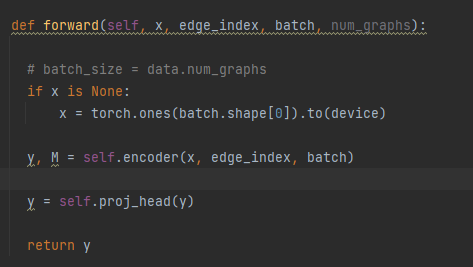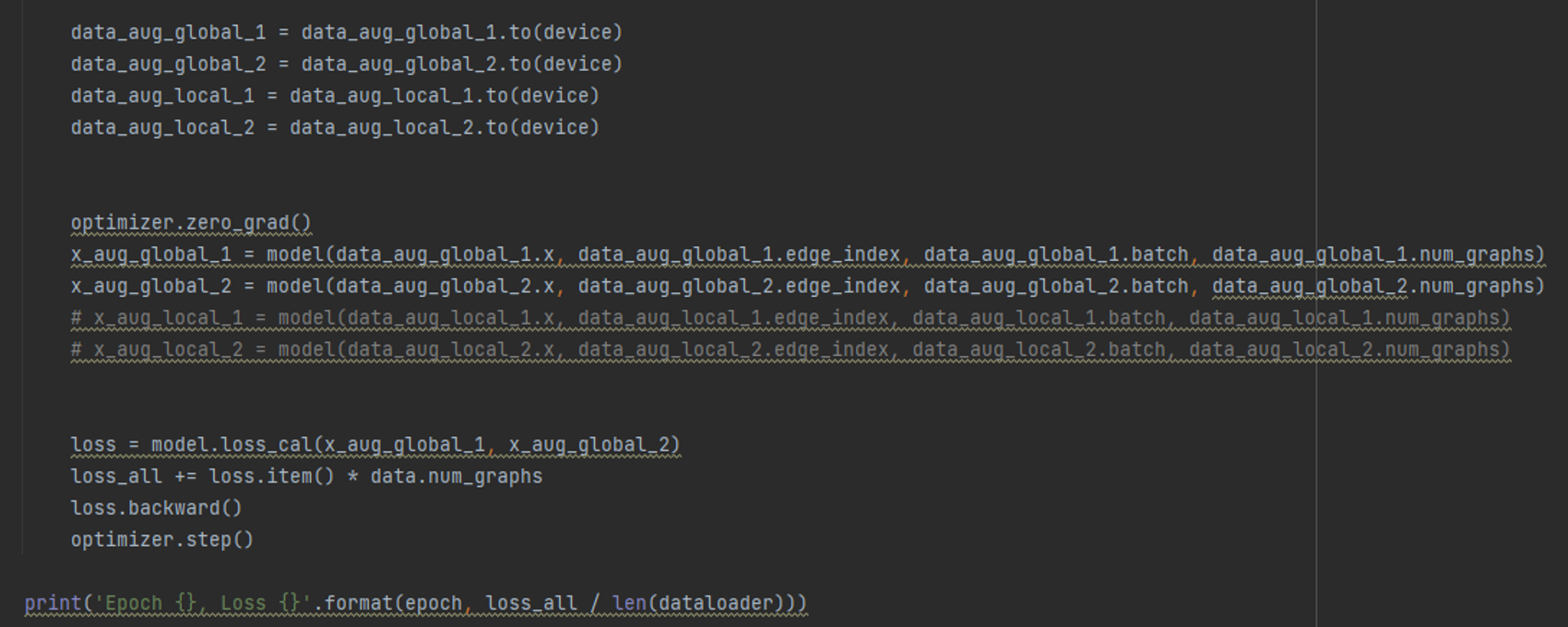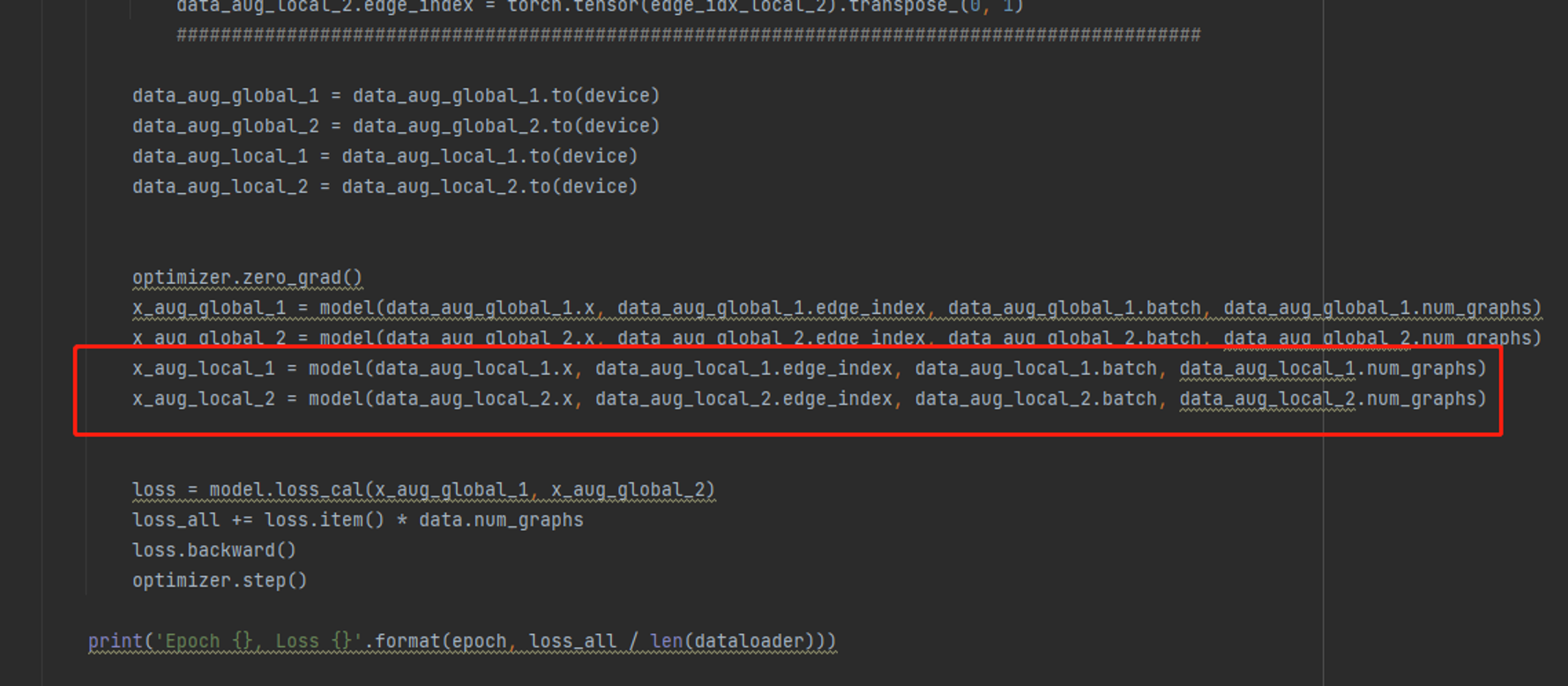### Environment

• PyG version:2.0.4
• PyTorch version:1.9
• OS:linux server
• Python version:3.8
• CUDA/cuDNN version:10.2
• How you installed PyTorch and PyG (`conda`, `pip`, source):pip
• Any other relevant information (e.g., version of `torch-scatter`):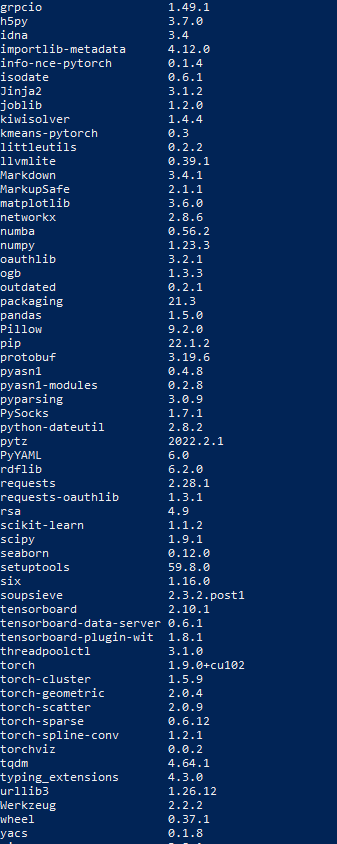bug
opened by ZhaoYuTJPU 9

### 🐛 Describe the bug

Context: I'm programmatically creating undirected graphs for training a Graph Autoencoder (GAE). Some graphs are very dense, containing 90% of all possible edges.

Training the GAE requires sampling missing edges as follows: `T.RandomLinkSplit(num_val=0.15, num_test=0, is_undirected=True, split_labels=True, add_negative_train_samples=True, neg_sampling_ratio=1.0)`

Issue: Because the graphs are too dense, there aren't enough missing edges to sample negative edges. Consider a graph with 100 nodes and 9000 edges (from a maximum of 9900 edges). There are only 900 missing edges to sample as negative edges, but because I'm setting `neg_sampling_ratio=1.0`, PyG expected to find 9000 missing edges.

The issue is that PyG doesn't check there aren't enough missing edges, and it creates training datasets without setting `neg_edge_label`. When creating the training data, the current code doesn't assign any `neg_edge_index` to the training data. See below the train/valid/test data.

``````(Data(x=[141, 141], edge_index=[2, 16072], pos_edge_label=, pos_edge_label_index=[2, 8036]), # Train; notice no negative edges
Data(x=[141, 141], edge_index=[2, 16072], pos_edge_label=, pos_edge_label_index=[2, 1417], neg_edge_label=, neg_edge_label_index=[2, 834]), # Validation
Data(x=[141, 141], edge_index=[2, 18906], pos_edge_label=, pos_edge_label_index=[2, 0])) # Test
``````

Error: When creating a dataloader comes the `KeyError: 'neg_edge_label'` because the training data is missing this key.

``````gnn_dataloader = tg.loader.DataLoader(graphs_dataset, batch_size=len(graphs_dataset), shuffle=True)
gnn_batch_train, _, _ = next(iter(gnn_dataloader))
``````
``````---------------------------------------------------------------------------
KeyError                                  Traceback (most recent call last)
/tmp/ipykernel_175984/1912375602.py in <module>
----> 2 gnn_batch_train, _, _ = next(iter(gnn_dataloader))

519             if self._sampler_iter is None:
520                 self._reset()
--> 521             data = self._next_data()
522             self._num_yielded += 1
523             if self._dataset_kind == _DatasetKind.Iterable and \

559     def _next_data(self):
560         index = self._next_index()  # may raise StopIteration
--> 561         data = self._dataset_fetcher.fetch(index)  # may raise StopIteration
562         if self._pin_memory:
563             data = _utils.pin_memory.pin_memory(data)

~/miniconda3/envs/low-data/lib/python3.7/site-packages/torch/utils/data/_utils/fetch.py in fetch(self, possibly_batched_index)
50         else:
51             data = self.dataset[possibly_batched_index]
---> 52         return self.collate_fn(data)

32             return type(elem)(*(self(s) for s in zip(*batch)))
33         elif isinstance(elem, Sequence) and not isinstance(elem, str):
---> 34             return [self(s) for s in zip(*batch)]
35
36         raise TypeError(f'DataLoader found invalid type: {type(elem)}')

32             return type(elem)(*(self(s) for s in zip(*batch)))
33         elif isinstance(elem, Sequence) and not isinstance(elem, str):
---> 34             return [self(s) for s in zip(*batch)]
35
36         raise TypeError(f'DataLoader found invalid type: {type(elem)}')

18         if isinstance(elem, BaseData):
19             return Batch.from_data_list(batch, self.follow_batch,
---> 20                                         self.exclude_keys)
21         elif isinstance(elem, torch.Tensor):
22             return default_collate(batch)

~/miniconda3/envs/low-data/lib/python3.7/site-packages/torch_geometric/data/batch.py in from_data_list(cls, data_list, follow_batch, exclude_keys)
72             add_batch=not isinstance(data_list, Batch),
73             follow_batch=follow_batch,
---> 74             exclude_keys=exclude_keys,
75         )
76

~/miniconda3/envs/low-data/lib/python3.7/site-packages/torch_geometric/data/collate.py in collate(cls, data_list, increment, add_batch, follow_batch, exclude_keys)
68                 continue
69
---> 70             values = [store[attr] for store in stores]
71
72             # The `num_nodes` attribute needs special treatment, as we need to

~/miniconda3/envs/low-data/lib/python3.7/site-packages/torch_geometric/data/collate.py in <listcomp>(.0)
68                 continue
69
---> 70             values = [store[attr] for store in stores]
71
72             # The `num_nodes` attribute needs special treatment, as we need to

~/miniconda3/envs/low-data/lib/python3.7/site-packages/torch_geometric/data/storage.py in __getitem__(self, key)
68
69     def __getitem__(self, key: str) -> Any:
---> 70         return self._mapping[key]
71
72     def __setitem__(self, key: str, value: Any):

KeyError: 'neg_edge_label'
``````

Suggested solution: I suggest a two-step solution:

1. Raise a Warning that there aren't enough missing edges to sample negative edges
2. If one of train/valid/test contains the keys `neg_edge_label` and `neg_edge_label_index`, then all three datasets shall contain it. In the above example, valid/test contain these keys, but the training dataset doesn't.

### Environment

• PyG version: 2.1.0
• PyTorch version: 1.10.1
• Python version: 3.7.9
bug transform
opened by andreimargeloiu 1
• #### Add Typehinting and checking for PyG Data and Batch #5172

As per #5172

Includes functionality to use torchtyping to check dimensions of tensors.

Looking for general feedback on implementation and style. Specifically, should we add required attributes for `Batch`es (like `ptr` and `batch`)?

The `dev.ipynb` notebook contains some examples that can be deleted or used as a starting point for documentation.

opened by a-r-j 1

### 😵 Describe the installation problem

I'm installing `pytorch_geometric` on apple M1. I use pip to install it on a conda environment.

`/opt/anaconda3/envs/MyEnv/bin/pip install torch-scatter torch-sparse torch-cluster torch-spline-conv torch-geometric -f https://data.pyg.org/whl/torch-1.12.0+cpu.html`

Installation is completed with no issue. Upon running

`import torch_geometric as tg`

I get the error

`Process finished with exit code 139 (interrupted by signal 11: SIGSEGV)`

### Environment

• PyG version: 1.12.0
• PyTorch version: Nightly
• OS: macOS 11.5.2 BigSur
• Python version: 3.7
• CUDA/cuDNN version: NA
• How you installed PyTorch and PyG (`conda`, `pip`, source): Both pip through conda, as shown above
• Any other relevant information (e.g., version of `torch-scatter`): NA
installation
opened by nshervt 1
##### Releases(2.1.0)
###### Matthias Fey
PhD student @ TU Dortmund University - Interested in Representation Learning on Graphs and Manifolds; PyTorch, CUDA, Vim and macOS Enthusiast###### A geometric deep learning pipeline for predicting protein interface contacts.

A geometric deep learning pipeline for predicting protein interface contacts.

43 Sep 1, 2022
###### Implementation of Geometric Vector Perceptron, a simple circuit for 3d rotation equivariance for learning over large biomolecules, in Pytorch. Idea proposed and accepted at ICLR 2021

Geometric Vector Perceptron Implementation of Geometric Vector Perceptron, a simple circuit with 3d rotation equivariance for learning over large biom

56 Sep 2, 2022
###### Code for "SRHEN: Stepwise-Refining Homography Estimation Network via Parsing Geometric Correspondences in Deep Latent Space"

SRHEN This is a better and simpler implementation for "SRHEN: Stepwise-Refining Homography Estimation Network via Parsing Geometric Correspondences in

0 Jan 18, 2022
###### Contains code for Deep Kernelized Dense Geometric Matching

DKM - Deep Kernelized Dense Geometric Matching Contains code for Deep Kernelized Dense Geometric Matching We provide pretrained models and code for ev

67 Sep 21, 2022
###### A PyTorch implementation of "DGC-Net: Dense Geometric Correspondence Network"

DGC-Net: Dense Geometric Correspondence Network This is a PyTorch implementation of our work "DGC-Net: Dense Geometric Correspondence Network" TL;DR A

188 Sep 28, 2022
553 Sep 22, 2022
###### TorchX: A PyTorch Extension Library for More Efficient Deep Learning

TorchX TorchX: A PyTorch Extension Library for More Efficient Deep Learning. @misc{torchx, author = {Ansheng You and Changxu Wang}, title = {T

8 May 28, 2022
###### Geometric Vector Perceptron --- a rotation-equivariant GNN for learning from biomolecular structure

Geometric Vector Perceptron Code to accompany Learning from Protein Structure with Geometric Vector Perceptrons by B Jing, S Eismann, P Suriana, RJL T

72 Sep 27, 2022
###### Geometric Vector Perceptrons --- a rotation-equivariant GNN for learning from biomolecular structure

Geometric Vector Perceptron Implementation of equivariant GVP-GNNs as described in Learning from Protein Structure with Geometric Vector Perceptrons b

123 Sep 23, 2022
###### Multi-Scale Geometric Consistency Guided Multi-View Stereo

ACMM [News] The code for ACMH is released!!! [News] The code for ACMP is released!!! About ACMM is a multi-scale geometric consistency guided multi-vi

112 Sep 16, 2022
###### Code for "FGR: Frustum-Aware Geometric Reasoning for Weakly Supervised 3D Vehicle Detection", ICRA 2021

FGR This repository contains the python implementation for paper "FGR: Frustum-Aware Geometric Reasoning for Weakly Supervised 3D Vehicle Detection"(I

30 Jun 1, 2022
###### Certifiable Outlier-Robust Geometric Perception

Certifiable Outlier-Robust Geometric Perception About This repository holds the implementation for certifiably solving outlier-robust geometric percep

77 Sep 26, 2022
###### 3DMV jointly combines RGB color and geometric information to perform 3D semantic segmentation of RGB-D scans.

3DMV 3DMV jointly combines RGB color and geometric information to perform 3D semantic segmentation of RGB-D scans. This work is based on our ECCV'18 p

0 Feb 6, 2022
###### Geometric Algebra package for JAX

JAXGA - JAX Geometric Algebra GitHub | Docs JAXGA is a Geometric Algebra package on top of JAX. It can handle high dimensional algebras by storing onl

8 Jul 22, 2022
###### Computational modelling of ray propagation through optical elements using the principles of geometric optics (Ray Tracer)

Computational modelling of ray propagation through optical elements using the principles of geometric optics (Ray Tracer) Introduction By applying the

1 Jul 9, 2022
###### GeoTransformer - Geometric Transformer for Fast and Robust Point Cloud Registration

Geometric Transformer for Fast and Robust Point Cloud Registration PyTorch imple

173 Sep 27, 2022
###### Buffon’s needle: one of the oldest problems in geometric probability

Buffon-s-Needle Buffon’s needle is one of the oldest problems in geometric proba

3 Feb 18, 2022
###### Implementation of GeoDiff: a Geometric Diffusion Model for Molecular Conformation Generation (ICLR 2022).

GeoDiff: a Geometric Diffusion Model for Molecular Conformation Generation [OpenReview] [arXiv] [Code] The official implementation of GeoDiff: A Geome

107 Sep 19, 2022
###### DeepProbLog is an extension of ProbLog that integrates Probabilistic Logic Programming with deep learning by introducing the neural predicate.

DeepProbLog DeepProbLog is an extension of ProbLog that integrates Probabilistic Logic Programming with deep learning by introducing the neural predic

79 Sep 8, 2022